Search Results
• #### find the transfer function G1(s)=θ1(s)/T(s), G3(s)=θ3(s)/T(s) for the following rotational mechanical system with gears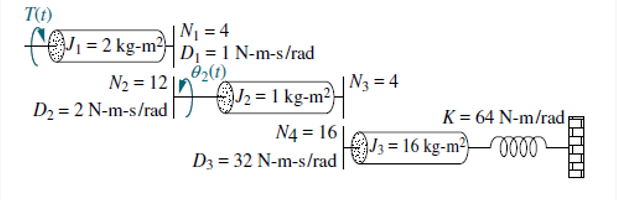This VI is use to find the polynomial of the transfer functions defined by G1(s)=θ1(s)/T(s), G2(s)=θ2(s)/T(s) and G3(s)=θ3(s)/T(s). Find the transfer function G1(s)=θ1(s)/T(s) and G3(s)=θ3(s)/T(s).

• #### question from analysis and design of control systems subject help please! R(s) s +1 Y(s) s(s+2)(s+20)...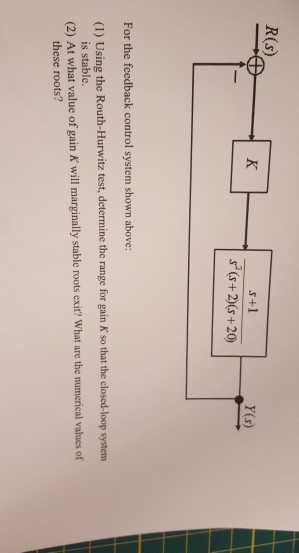question from analysis and design of control systems subject help please! R(s) s +1 Y(s) s(s+2)(s+20) For the feedback control system shown above (I) Using the Routh-Hurwitz test, determine the range for gain K so that the closed-loop system (2) At wha is stable. t value of gain K will marginally stable roots exiť? What are the numerical values of...

• #### 3. Assume G(s) . For the unity negative feedback system shown below: (s-2)(s-4) R(s)+ C(s) G(s)...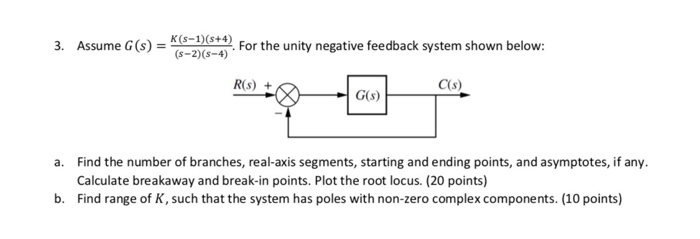3. Assume G(s) . For the unity negative feedback system shown below: (s-2)(s-4) R(s)+ C(s) G(s) a. Find the number of branches, real-axis segments, starting and ending points, and asymptotes, if any Calculate breakaway and break-in points. Plot the root locus. (20 points) Find range of K, such that the system has poles with non-zero complex components.(10 points) b.

• #### plot Bode diagrams of Gi(s) and G2(s) given below. 1 + S G(s)12s G2(s)1 G1(s) is a minimum-phase system and G2(s) is a...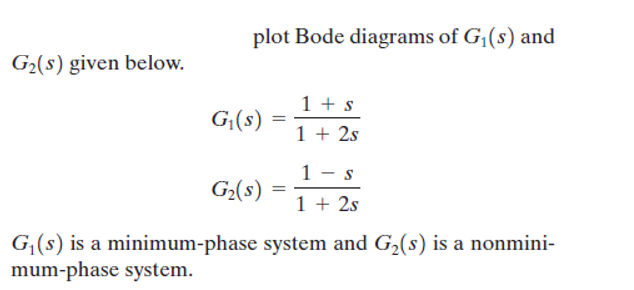plot Bode diagrams of Gi(s) and G2(s) given below. 1 + S G(s)12s G2(s)1 G1(s) is a minimum-phase system and G2(s) is a nonmini- mum-phase system. plot Bode diagrams of Gi(s) and G2(s) given below. 1 + S G(s)12s G2(s)1 G1(s) is a minimum-phase system and G2(s) is a nonmini- mum-phase system.

• #### Y(s) s216 s 128 (s212 s 52) (s +1) (s + 4) (s +8) R(s) For the given system above, Sketch the root locus. Procedures Sh...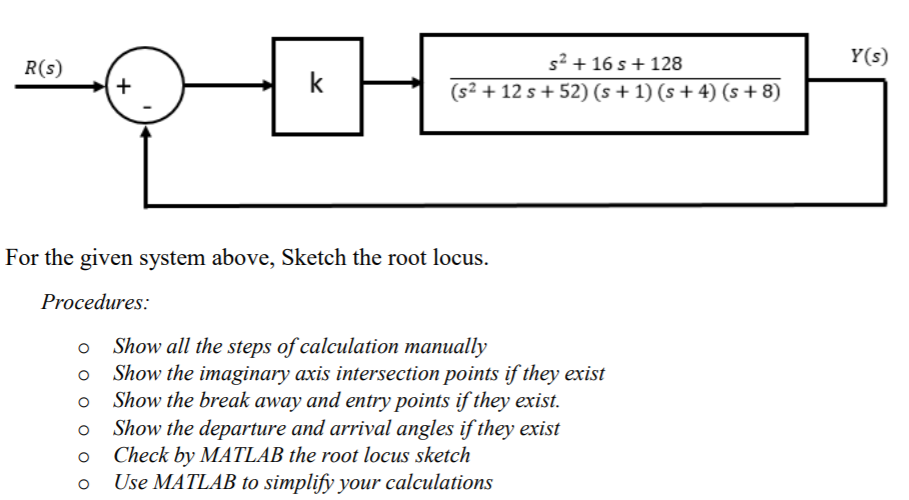Y(s) s216 s 128 (s212 s 52) (s +1) (s + 4) (s +8) R(s) For the given system above, Sketch the root locus. Procedures Show all the steps of calculation manually Show the imaginary axis intersection points if they exist Show the break away and entry points if they exist. Show the departure and arrival angles if they exist...

• #### Figure 1 shows a closed-loop control system in which G(S)=40/[ (S+2) (S+3)], and H(S)=1/(S+4) R(S) E(S)...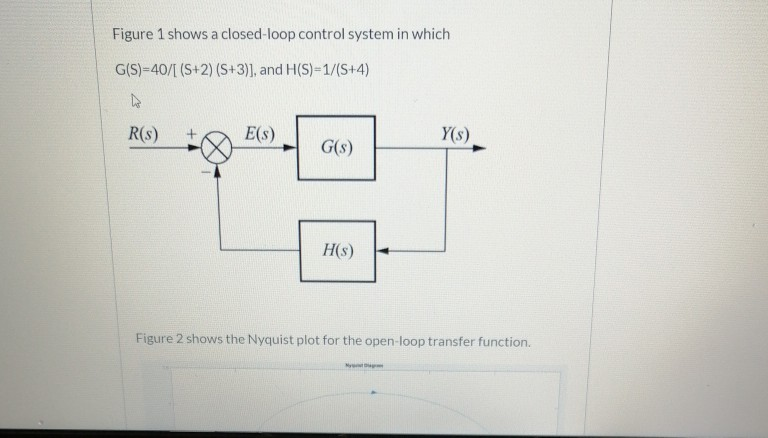Figure 1 shows a closed-loop control system in which G(S)=40/[ (S+2) (S+3)], and H(S)=1/(S+4) R(S) E(S) Y(s) G(S) HS) Figure 2 shows the Nyquist plot for the open-loop transfer function. Figure 2 shows the Nyquist plot for the open-loop transfer function System: sys Real: -0.187 Imag: 2.56e-05 Frequency: (rad/s): -5.16 Using the Nyquist criterion: a) Find out the gain margin...

• #### 3. Consider the tilt control block diagram shown below R(s) DesiredG(s) 12 s(s+10)(s+70) Y(s) Til...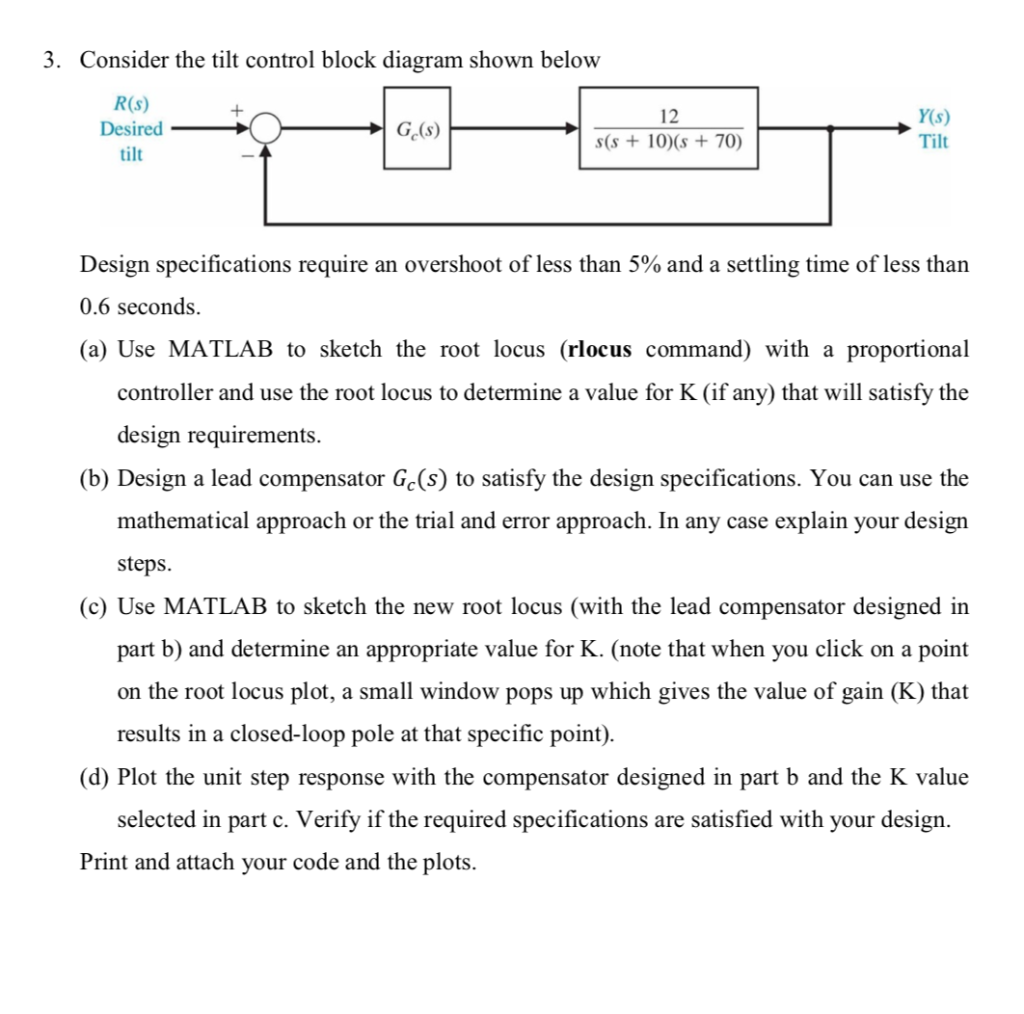3. Consider the tilt control block diagram shown below R(s) DesiredG(s) 12 s(s+10)(s+70) Y(s) Tilt tilt Design specifications require an overshoot of less than 5% and a settling time of less than 0.6 seconds. (a) Use MATLAB to sketch the root locus (rlocus command) with a proportional controller and use the root locus to determine a value for K (if...

• #### Explain why S is not a basis for M2,2 s-1 1 S is linearly dependent S does not span M2,2 S is lin...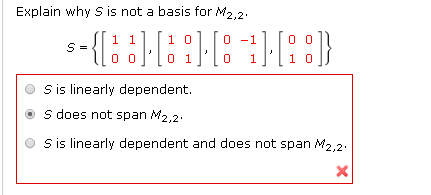Explain why S is not a basis for M2,2 s-1 1 S is linearly dependent S does not span M2,2 S is linearly dependent and does not span M2,2 Explain why S is not a basis for M2,2 s-1 1 S is linearly dependent S does not span M2,2 S is linearly dependent and does not span M2,2

• #### Find the Bode diagram of the following systems (Points: 25): 100( S1) G(S)S(S+1){ (S)+ S+ 1...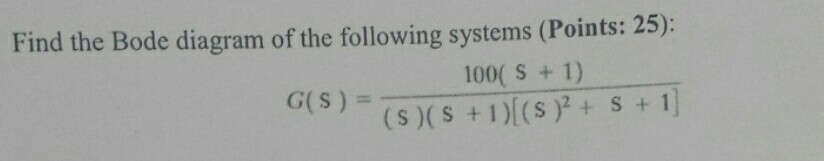Find the Bode diagram of the following systems (Points: 25): 100( S1) G(S)S(S+1){ (S)+ S+ 1 Find the Bode diagram of the following systems (Points: 25): 100( S1) G(S)S(S+1){ (S)+ S+ 1